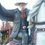# Work Inspired by a wiki page:

This wiki page page inspired me to do discover $$\sum^n_{i=1}i^a$$ with $$a$$ being a natural number; I will just start using $$\sum^n_{i=1}i^0=1^0+2^0+3^0+...+n^0=n$$ so I can discover the next ones; Using the same method that the linked page had done with $$n^2$$ and $$n^3$$, I will discover the polynomial that is equal to $$\sum^n_{i=1}i^a$$;

First, I will use binomial theorem:

$(n+1)^{a+1}=\sum^{a+1}_{i=0}\binom{a+1}{i}n^i$ The $1^{a+1-i}$ will be always 1, so it will not change the result if not written

Therefore:

$(n+1)^{a+1}=n^{a+1}+\sum^a_{i=0}\binom{a+1}{i}n^i$ (I took the "$n^{a+1}$" out of the sum notation)

$(n+1)^{a+1}-n^{a+1}=\sum^a_{i=0}\binom{a+1}{i}n^i$

As the page did, I will substitute n by 1,2,3....n

$2^{a+1}-1^{a+1}=\sum^a_{i=0}\binom{a+1}{i}1^i$

$3^{a+1}-2^{a+1}=\sum^a_{i=0}\binom{a+1}{i}2^i$

$4^{a+1}-3^{a+1}=\sum^a_{i=0}\binom{a+1}{i}3^i$

$\vdots$

$n^{a+1}-(n-1)^{a+1}=\sum^a_{i=0}\binom{a+1}{i}(n-1)^i$

$(n+1)^{a+1}-n^{a+1}=\sum^a_{i=0}\binom{a+1}{i}n^i$

Then I will sum everything, notice that everything on the left side, except $(n+1)^{a+1}$ and $-1^{a+1}$ cancels themselves, for the right side, every sum has now another sum inside it!

$(n+1)^{a+1}-1=\sum^a_{i=0}\left (\binom{a+1}{i}\sum^n_{j=1}j^i \right )$

I will work the left side now, remember what $(n+1)^{a+1}$ is, but now, we will have to take the $\binom{a+1}{0}n^0=1$, because it will cancel with $-1$:

$\sum^{a+1}_{i=1}\binom{a+1}{i}n^i=\sum^a_{i=0}\left (\binom{a+1}{i}\sum^n_{j=1}j^i \right )$

Now, I will take the term that appears on the sum of the right when i=a out of the notation:

$\sum^{a+1}_{i=1}\binom{a+1}{i}n^i=\binom{a+1}{a}\sum^n_{j=1}j^a+\sum^{a-1}_{i=0}\left (\binom{a+1}{i}\sum^n_{j=1}j^i \right )$

Notice that this term is exactally what we are searching, so I will isolate it:

$\binom{a+1}{a}\sum^n_{j=1}j^a=\sum^{a+1}_{i=1}\binom{a+1}{i}n^i-\sum^{a-1}_{i=0}\left (\binom{a+1}{i}\sum^n_{j=1}j^i \right )$

Remember that $\binom{a+1}{a}=\binom{a+1}{1}=a+1$:

$\sum^n_{j=1}j^a=\frac{\sum^{a+1}_{i=1}\binom{a+1}{i}n^i-\sum^{a-1}_{i=0}\left (\binom{a+1}{i}\sum^n_{j=1}j^i \right )}{a+1}$

Now, Remember that, when $a=0$, we have a polynomial expression $n$, then if $a=2$ also "is"(As in: there is a polynomial expression that has the same values) a polynomial expression, and, by a strong induction, if a is natural, then the expression $\sum^n_{i=1}i^a$ also "is".

I also made a program, in Python, that returned the expression of $\sum^n_{i=1}i^a$, but to much more values other than 0, 1, 2, 3(Which the original page did), some of the results are:

$\sum^n_{i=1}i^{1}=\frac{n^2 + n}{2}$

$\sum^n_{i=1}i^{2}=\frac{2n^3 + 3n^2 + n}{6}$

$\sum^n_{i=1}i^{3}=\frac{n^4 + 2n^3 + n^{2}}{4}$

$\sum^n_{i=1}i^{4}=\frac{6n^5 + 15n^4 + 10n^3 - n}{30}$

$\sum^n_{i=1}i^{5}=\frac{2n^6 + 6n^5 + 5n^4 - 1n^2}{12}$

$\sum^n_{i=1}i^{6}=\frac{6n^7 + 21n^6 + 21n^5 - 7n^3 + n}{42}$

$\sum^n_{i=1}i^{7}=\frac{3n^{8} + 12n^{7} + 14n^{6} - 7n^{4} + 2n^{2}}{24}$

$\sum^n_{i=1}i^{8}=\frac{10n^{9} + 45n^{8} + 60n^{7} - 42n^{5} + 20n^{3} - 3n}{90}$

$\sum^n_{i=1}i^{9}=\frac{2n^{10} + 10n^{9} + 15n^{8} - 14n^{6} + 10n^{4} - 3n^{2}}{20}$

There are patterns there, but I will make another note to that, as this is getting hugeNote by Matheus Jahnke
4 years, 7 months ago

This discussion board is a place to discuss our Daily Challenges and the math and science related to those challenges. Explanations are more than just a solution — they should explain the steps and thinking strategies that you used to obtain the solution. Comments should further the discussion of math and science.

When posting on Brilliant:

• Use the emojis to react to an explanation, whether you're congratulating a job well done , or just really confused .
• Ask specific questions about the challenge or the steps in somebody's explanation. Well-posed questions can add a lot to the discussion, but posting "I don't understand!" doesn't help anyone.
• Try to contribute something new to the discussion, whether it is an extension, generalization or other idea related to the challenge.

MarkdownAppears as
*italics* or _italics_ italics
**bold** or __bold__ bold
- bulleted- list
• bulleted
• list
1. numbered2. list
1. numbered
2. list
Note: you must add a full line of space before and after lists for them to show up correctly
paragraph 1paragraph 2

paragraph 1

paragraph 2

[example link](https://brilliant.org)example link
> This is a quote
This is a quote
    # I indented these lines
# 4 spaces, and now they show
# up as a code block.

print "hello world"
# I indented these lines
# 4 spaces, and now they show
# up as a code block.

print "hello world"
MathAppears as
Remember to wrap math in $$ ... $$ or $ ... $ to ensure proper formatting.
2 \times 3 $2 \times 3$
2^{34} $2^{34}$
a_{i-1} $a_{i-1}$
\frac{2}{3} $\frac{2}{3}$
\sqrt{2} $\sqrt{2}$
\sum_{i=1}^3 $\sum_{i=1}^3$
\sin \theta $\sin \theta$
\boxed{123} $\boxed{123}$

Sort by:

This is amazing, i never knew we can generalize it !! Before reading this note , i used to approximate the answer.

$S_{k} = 1^{k}+2^{k}+3^{k}+\cdots+n^{k} =\displaystyle\sum_{r=1}^{n} r^{k}$

For a large value of $n$ (probably greater than $1000$ for negligible error) we can convert the sum in an integral

$\displaystyle\sum_{r=0}^{n}r^{k} = n^{k+1} \int_{0}^{1} x^{k} dx \\ S_{k} \approx \dfrac{n^{k+1}}{k+1}$

For example take $k =1$ and $n = 2000$

$S_{1} \approx \dfrac{2000^{2}}{2} = 2000000 \\ \text{exact sum = } 2001000$

an error of only $1000$ . the bigger the value of $n$ , more accurate is the approximation

- 4 years, 7 months ago

That is one of the patterns:

The term with the highest degree of the equivalent of $\sum^n_{i=1}i^a$ is $\frac{n^{a+1}}{a+1}$

We can prove it by induction, as in: it works for $a = 1$, because $\sum^n_{i=1} i^1=\frac{n^2}{1+1} + ...$

If it works for $a = k, k-1,k-2, ...$, then for $a = k + 1$, we have:

$\sum^n_{i=1}i^{k+1}=\frac{\sum^{k+2}_{i=1}\binom{k+2}{i}n^i-\left(\sum^{a}_{i=0}\binom{k+2}{i}\sum^n_{j=1}j^i\right )}{k+2}$

The term with the highest degree is $\frac{n^{(k+1)+1}}{(k+1)+1}$ due the first sum highest degree, no term of the other sum has a equal or higher degree, because the highest one is $\sum^n_{i=1}i^k$, which gives $\frac{n^{k+1}}{k+1}$

And as the others sums have a lower exponent, then they will have a lower degree of term(this is a strong induction)

- 4 years, 7 months ago

There's a (somewhat) general result for this involving Bernoulli numbers. It's called Faulhaber's formula.

- 4 years, 7 months ago

That is a very good comment, thanks for linking it, even though it make everything I did useless...

- 4 years, 7 months ago